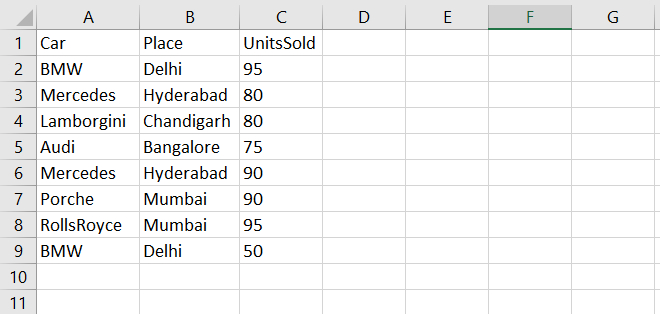# How to do groupby on a multiindex in Pandas?

PythonServer Side ProgrammingProgramming

Multiindex Data Frame is a data frame with more than one index. Let’s say the following is our csv stored on the Desktop −At first, import the pandas library and read the above CSV file −

import pandas as pd

df = pd.read_csv("C:/Users/amit_/Desktop/sales.csv") print(df)

We will form the ‘Car‘ and ‘Place‘ columns of the Dataframe as the index −

df = df.set_index(['Car', 'Place'])

The DataFrame is now a multi-indexed DataFrame having the ‘Car‘ and ‘Place‘ columns as an index.

Now, let us use groupby on the multiindex dataframe:

res = df.groupby(level=['Car'])['UnitsSold'].mean() print(res)

## Example

Following is the code −

import pandas as pd

print(df)

# set Car and Place columns of the DataFrame as index
df = df.set_index(['Car', 'Place'])

# sorting
df.sort_index()

# groupby on multiindex datafram
res = df.groupby(level=['Car'])['UnitsSold'].mean()
print(res)

## Output

This will produce the following output −

          Car         Place       Sold
0         BMW         Delhi         95
2  Lamborgini    Chandigarh         80
3        Audi     Bangalore         75
Name: UnitsSold, dtype: float64